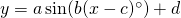# Apply the Sinusoidal Function

The objective of this short project is to use apply knowledge and skills of trigonometric functions to a real life context.

There are four areas of inquiry suggested in the table below. Each area of inquiry will yield a graph that is periodic and has very similar properties to the sine (or cosine) curve. Therefore an equation of the formwill be a good choice of curve to model the data.

Choose one area of inquiry from the four listed below, or pursue your own. Using the values in the data set to calculate parameters a and d, and using the period of the curve to calculate b and if necessary c, find an equation of the form that models the data well.

Once your model is made, what can it be used for? In what way or ways does the real life situation differ from your model? Can your model be improved in any way?

This is an exercise in calculating the parameters a, b, c and d.  I may be interesting to see how your curve compares to one generated by geogebra (‘fitsin’ command); or desmos (regression).

Note that data can often be imported directly by copy, paste to geogebra spreadsheet/desmos table, or via an excel spreadsheet/google sheet.

Graphing on geogebra classic.  Graphing on desmos.